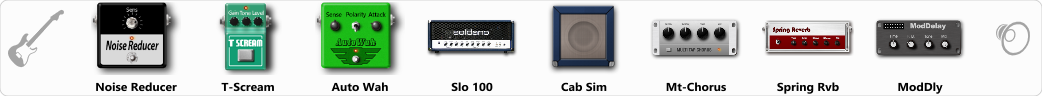Discussion in 'ToneLib-GFX presets' started by pkurg, Aug 14, 2019.

Related video:

Effects chain:Effect: "Noise Reducer" (Dynamics / Filter), active - "yes"
"Sens" = 100
"Mode" = Soft

Effect: "T-Scream" (Overdrive / Distortion), active - "yes"
"Drive" = 23
"Tone" = 79
"Level" = 79

Effect: "Auto Wah" (Dynamics / Filter), active - "yes"
"Sense" = 50
"Polarity" = Up
"Attack" = 42
"Style" = V848

Effect: "Slo 100" (Amp simulators), active - "yes"
"Gain" = 100
"Bass" = 40
"Middle" = 57
"Treble" = 35
"Presence" = 30
"Master" = 69
"Output" = 50
"Level (dB)" = 0

Effect: "Cab Sim" (Cabinets), active - "yes"
"Model" = 4x12" 1960 T75
"Level (dB)" = 0

Effect: "Mt-Chorus" (Modulation / Sfx), active - "yes"
"Speed" = 3.1
"Depth" = 69
"Time" = 3.4
"Mix" = 61

Effect: "Spring Rvb" (Reverb), active - "yes"
"Time" = 7.0
"PreDelay" = 0
"LoDamp" = 16
"HiDamp" = 26
"Mix" = 42

Effect: "ModDly" (Delay), active - "yes"
"Time" = 648
"Feedback" = 39
"Tone" = 70
"Speed" = 1.7
"Mix" = 50

Note: You will need to download and install the ToneLib-GFX software to use the preset.